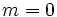# General affine group:GA(1,5)

View a complete list of particular groups (this is a very huge list!)[SHOW MORE]

## Definition

This group is defined in the following equivalent ways:

1. It is the general affine group of degree one over the field of five elements. In other words, it is the semidirect product of the additive and multiplicative groups of this field. It is denoted$GA(1,5)$.
2. It is the holomorph of the cyclic group of order five.
3. It is the Suzuki group$Sz(2)$ or the Suzuki group$Sz(2^{1 + 2m})$ where$m = 0$. Note: This is the only non-simple Suzuki group.

The group can be given by the presentation, with$e$ denoting the identity element:$\langle a,b \mid a^5 = b^4 = e, bab^{-1} = a^2 \rangle$

## Arithmetic functions

Function Value Similar groups Explanation
order (number of elements, equivalently, cardinality or size of underlying set) 20 groups with same order As$GA(1,q)$,$q = 5$:$q(q - 1) = 5(5 - 1) = 20$
As holomorph of cyclic group:Z5:$|\mathbb{Z}_5||\operatorname{Aut}(\mathbb{Z}_5)| = 5 \cdot 4 = 20$
As$\! Sz(q), q = 2$:$q^2(q^2 + 1)(q - 1) = 2^2(2^2 + 1)(2 - 1) = 4 \cdot 5 \cdot 1 = 20$
exponent of a group 20 groups with same order and exponent of a group | groups with same exponent of a group The subgroup of order five has an element of order five; the acting subgroup of order four is cyclic and has an element of order four.
Frattini length 1 groups with same order and Frattini length | groups with same Frattini length
Fitting length 2 groups with same order and Fitting length | groups with same Fitting length
derived length 2 groups with same order and derived length | groups with same derived length
minimum size of generating set 2 groups with same order and minimum size of generating set | groups with same minimum size of generating set Generator of cyclic group of order five, generator of acting cyclic group of order four.
subgroup rank of a group 2 groups with same order and subgroup rank of a group | groups with same subgroup rank of a group

## Group properties

Function Value Explanation
abelian group No
nilpotent group No
metacyclic group Yes
supersolvable group Yes
solvable group Yes
Frobenius group Yes
Camina group Yes

## GAP implementation

### Group ID

This finite group has order 20 and has ID 3 among the groups of order 20 in GAP's SmallGroup library. For context, there are 5 groups of order 20. It can thus be defined using GAP's SmallGroup function as:

SmallGroup(20,3)

For instance, we can use the following assignment in GAP to create the group and name it$G$:

gap> G := SmallGroup(20,3);

Conversely, to check whether a given group$G$ is in fact the group we want, we can use GAP's IdGroup function:

IdGroup(G) = [20,3]

or just do:

IdGroup(G)

to have GAP output the group ID, that we can then compare to what we want.